# Characteristic Vector for T3 Coordinates

Let's apply Jay's Characteristic Vector approach to Joel's T3 Coordinate System.

## Brute Force Manipulations

Starting from Equation CV.02, and plugging in expressions for various logarithmic derivatives of the T3 scale factors, we obtain [Note: Sign error from equation CV.02 fixed here on 15 July 2010],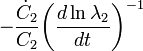$- \frac{\dot{C}_2}{C_2} \biggl(\frac{d \ln{\lambda}_2}{dt}\biggr)^{-1}$ =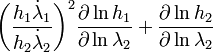$\biggl(\frac{h_1 \dot{\lambda}_1}{h_2 \dot{\lambda}_2}\biggr)^2 \frac{\partial \ln h_1}{\partial\ln\lambda_2} + \frac{\partial \ln h_2}{\partial \ln\lambda_2}$ =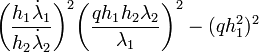$\biggl(\frac{h_1 \dot{\lambda}_1}{h_2 \dot{\lambda}_2}\biggr)^2 \biggl( \frac{q h_1 h_2 \lambda_2}{\lambda_1 } \biggr)^2 - ( qh_1^2 )^2$ =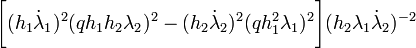$\biggl[ (h_1 \dot{\lambda}_1)^2 ( q h_1 h_2 \lambda_2 )^2 - (h_2 \dot{\lambda}_2)^{2} ( qh_1^2 \lambda_1 )^2 \biggr](h_2 \lambda_1 \dot{\lambda}_2)^{-2}$ =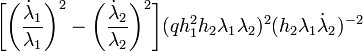$\biggl[ \biggl(\frac{\dot{\lambda}_1}{\lambda_1}\biggr)^2 - \biggl( \frac{\dot{\lambda}_2}{\lambda_2} \biggr)^2 \biggr]( q h_1^2 h_2 \lambda_1 \lambda_2 )^2 (h_2 \lambda_1 \dot{\lambda}_2)^{-2}$ =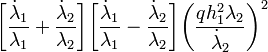$\biggl[ \frac{\dot{\lambda}_1}{\lambda_1} + \frac{\dot{\lambda}_2}{\lambda_2} \biggr] \biggl[ \frac{\dot{\lambda}_1}{\lambda_1} - \frac{\dot{\lambda}_2}{\lambda_2} \biggr] \biggl( \frac{ q h_1^2 \lambda_2}{\dot{\lambda}_2} \biggr)^2$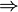$\Rightarrow$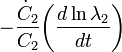$- \frac{\dot{C}_2}{C_2} \biggl(\frac{d \ln{\lambda}_2}{dt}\biggr)$ =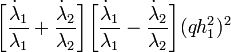$\biggl[ \frac{\dot{\lambda}_1}{\lambda_1} + \frac{\dot{\lambda}_2}{\lambda_2} \biggr] \biggl[ \frac{\dot{\lambda}_1}{\lambda_1} - \frac{\dot{\lambda}_2}{\lambda_2} \biggr] ( q h_1^2 )^2$ =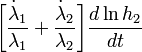$\biggl[ \frac{\dot{\lambda}_1}{\lambda_1} + \frac{\dot{\lambda}_2}{\lambda_2} \biggr] \frac{d\ln h_2}{dt}$ =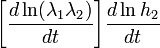$\biggl[ \frac{d\ln(\lambda_1 \lambda_2)}{dt} \biggr] \frac{d\ln h_2}{dt}$

## Two Views of Equation of Motion

### Christoffel Symbol Formalism

The second component of the equation of motion can be obtained by setting i = 2 and Ci = 1 in Equation CV.01, specifically,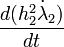$\frac{d(h_2^2 \dot{\lambda}_2)}{dt}$ =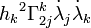${h_k}^2 \Gamma^k_{2j} \dot{\lambda}_j \dot{\lambda}_k$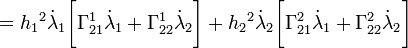$= {h_1}^2 \dot{\lambda}_1 \biggr[ \Gamma^1_{21} \dot{\lambda}_1 + \Gamma^1_{22} \dot{\lambda}_2 \biggl] + {h_2}^2 \dot{\lambda}_2 \biggr[ \Gamma^2_{21} \dot{\lambda}_1 + \Gamma^2_{22} \dot{\lambda}_2 \biggl]$ =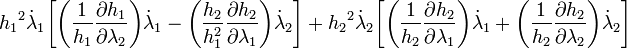${h_1}^2 \dot{\lambda}_1 \biggr[ \biggl( \frac{1}{h_1} \frac{\partial h_1}{\partial\lambda_2} \biggr) \dot{\lambda}_1 - \biggl( \frac{h_2}{h_1^2} \frac{\partial h_2}{\partial\lambda_1} \biggr) \dot{\lambda}_2 \biggl] + {h_2}^2 \dot{\lambda}_2 \biggr[ \biggl( \frac{1}{h_2} \frac{\partial h_2}{\partial\lambda_1} \biggr) \dot{\lambda}_1 + \biggl( \frac{1}{h_2} \frac{\partial h_2}{\partial\lambda_2} \biggr) \dot{\lambda}_2 \biggl]$ =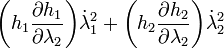$\biggl( h_1 \frac{\partial h_1}{\partial\lambda_2} \biggr) \dot{\lambda}_1^2 + \biggl( h_2 \frac{\partial h_2}{\partial\lambda_2} \biggr) \dot{\lambda}_2^2$

### Binney and Tremaine Formalism

We have also derived the second component of the equation of motion following the formalism outlined by Binney and Tremaine (BT87). Specifically, in our introductory discussion of the T3 Coordinate System our Equation EOM.01 has the form,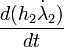$\frac{d(h_2 \dot{\lambda}_2)}{dt}$ =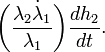$\biggl(\frac{\lambda_2 \dot{\lambda}_1}{\lambda_1}\biggr) \frac{dh_2}{dt} .$

To compare this with the form derived using the Christoffel symbol formalism, we need to multiply through by h2 and bring the scale factor inside the time-derivative on the left-hand-side.$\frac{d(h_2^2 \dot{\lambda}_2)}{dt}$ =$\biggl[ \biggl(\frac{h_2 \lambda_2 \dot{\lambda}_1}{\lambda_1}\biggr) + (h_2 \dot{\lambda}_2) \biggr]\frac{dh_2}{dt}$ =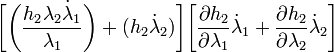$\biggl[ \biggl(\frac{h_2 \lambda_2 \dot{\lambda}_1}{\lambda_1}\biggr) + (h_2 \dot{\lambda}_2) \biggr]\biggl[ \frac{\partial h_2}{\partial\lambda_1} \dot{\lambda}_1 + \frac{\partial h_2}{\partial\lambda_2} \dot{\lambda}_2 \biggr]$ =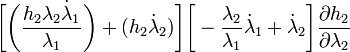$\biggl[ \biggl(\frac{h_2 \lambda_2 \dot{\lambda}_1}{\lambda_1}\biggr) + (h_2 \dot{\lambda}_2) \biggr]\biggl[ - \frac{\lambda_2}{\lambda_1} \dot{\lambda}_1 + \dot{\lambda}_2 \biggr] \frac{\partial h_2}{\partial\lambda_2}$ =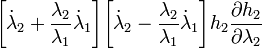$\biggl[ \dot{\lambda}_2 + \frac{\lambda_2 }{\lambda_1} \dot{\lambda}_1 \biggr]\biggl[ \dot{\lambda}_2 - \frac{\lambda_2}{\lambda_1} \dot{\lambda}_1 \biggr] h_2 \frac{\partial h_2}{\partial\lambda_2}$ =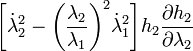$\biggl[ \dot{\lambda}_2^2 - \biggl( \frac{\lambda_2 }{\lambda_1}\biggr)^2 \dot{\lambda}_1^2 \biggr] h_2 \frac{\partial h_2}{\partial\lambda_2}$ =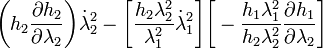$\biggl( h_2 \frac{\partial h_2}{\partial\lambda_2}\biggr) \dot{\lambda}_2^2 - \biggl[\frac{h_2 \lambda_2^2}{\lambda_1^2} \dot{\lambda}_1^2 \biggr] \biggl[- \frac{h_1 \lambda_1^2}{h_2 \lambda_2^2} \frac{\partial h_1}{\partial\lambda_2} \biggr]$ =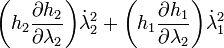$\biggl( h_2 \frac{\partial h_2}{\partial\lambda_2}\biggr) \dot{\lambda}_2^2 + \biggl( h_1 \frac{\partial h_1}{\partial\lambda_2}\biggr) \dot{\lambda}_1^2$

### Summary

So we see that, indeed, the two formalisms produce identical forms of the equation of motion.

## Implications

Backing up to the expression that began our examination of the Binney and Tremaine formalism, we also can write,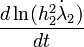$\frac{d\ln(h_2^2 \dot{\lambda}_2)}{dt}$ =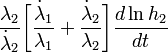$\frac{\lambda_2}{\dot{\lambda}_2} \biggl[ \frac{\dot{\lambda}_1}{\lambda_1} + \frac{\dot{\lambda}_2}{\lambda_2} \biggr]\frac{d\ln h_2}{dt}$$\Rightarrow$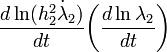$\frac{d\ln(h_2^2 \dot{\lambda}_2)}{dt} \biggl(\frac{d\ln\lambda_2}{dt}\biggr)$ =$\biggl[ \frac{d\ln(\lambda_1 \lambda_2)}{dt} \biggr]\frac{d\ln h_2}{dt}$

 NOTE:The following few boxed-in sentences/expressions are incorrect. They originally appeared in this discussion due to confusion that arose in conjunction with a sign error in the expression for dlnC2 / dt (see the top of this page). On 14 July 2010, following a lengthy discussion between Joel and Jay of the statements colored in green, Jay spotted the sign error. (See his 14 July 2010 talk-page comment.) The proper derivation/conclusion resulting from the corrected sign error follows these boxed-in sentences/expressions. Comparing this with the brute force derivation of the condition derived above for the characteristic vector, C2, we see that the two expressions are the same if we set,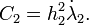$C_2 = h_2^2 \dot{\lambda}_2 .$ This seems to imply that we have discovered a conserved quantity, namely,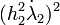$(h_2^2 \dot{\lambda}_2)^2$. On the other hand, I might just be using a circular argument; I might only be saying that "the equation of motion is the equation of motion!" Temp note (from Jay): Joel, I don't quite understand this. Next time we get together, can you explain this page to me?

Comparing this last differential equation with the brute force derivation of the condition derived above for the characteristic vector, C2, we see that the two expressions are the same if we set,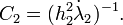$C_2 = ( h_2^2 \dot{\lambda}_2 )^{-1} .$

At first sight, this seems to imply that we have discovered a conserved quantity. But, alas, the result is a trivial one: The resulting conserved quantity is,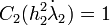$C_2(h_2^2 \dot{\lambda}_2) = 1$.

## Conserved Quantity

Let's cut to the chase. As shown on the page describing the characteristic vector approach, I can write down the third conserved quantity right now--just not in closed form. Assuming there's no potential variation in the direction of λ2, it is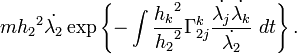$m{h_2}^2 \dot{\lambda_2} \exp \left\{ - \int \frac{{h_k}^2}{{h_2}^2} \Gamma^k_{2j} \frac{\dot{\lambda_j} \dot{\lambda_k}}{\dot{\lambda_2}} \ dt \right\} .$

In the case of T3 coordinates, this becomes more specific.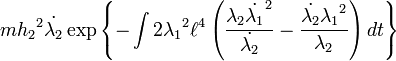$m {h_2}^2 \dot{\lambda_2} \exp \left\{ - \int 2 {\lambda_1}^2 \ell^4 \left( \frac{\lambda_2 \dot{\lambda_1}^2}{\dot{\lambda_2}} - \frac{\dot{\lambda_2}{\lambda_1}^2}{\lambda_2} \right) dt \right\}$

Although this is not all that useful in an analytic sense until we can integrate it, I wonder if it can be a guide to building a more accurate numerical model. Certainly this function can be integrated numerically, and that's got to be useful somehow...

## Thoughts on Integrating This Conserved Quantity

The quantity appearing inside the parentheses has an interesting symmetry. Each variable appearing without a dot in the first term appears in the same place with a dot in the second term, and vice versa. Certainly there must be some differentiation rule that will allow us to express this quantity as a total time derivative.

On the other hand, the factor of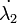$\dot{\lambda_2}$ appearing in the denominator of the first term is troublesome. I can't think of any differentiation rule that puts a derivative in the denominator. Product rule, quotient rule, and chain rule all end up multiplying by derivatives. So I wonder if there's some way to eliminate the$\dot{\lambda_2}$ in favor of undotted variables. This would require transforming the equation of motion for the$\dot{\lambda_2}$ coordinate into a first-order equation. Right now, the second-order equation reads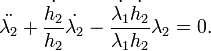$\ddot{\lambda_2} + \frac{\dot{h_2}}{h_2} \dot{\lambda_2} - \frac{\dot{\lambda_1} \dot{h_2}}{\lambda_1 h_2} \lambda_2 = 0 .$

The first step in reducing this to a first-order equation is to perform a transformation of variables that eliminates that$\dot{\lambda_2}$ term. I have successfully accomplished this. By defining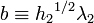$b \equiv {h_2}^{1/2} \lambda_2$, the equation can be written: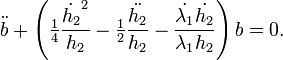$\ddot{b} + \left( \tfrac{1}{4} \frac{{\dot{h_2}}^2}{h_2} - \tfrac{1}{2} \frac{\ddot{h_2}}{h_2} - \frac{\dot{\lambda_1} \dot{h_2}}{\lambda_1 h_2} \right) b = 0 .$

 © 2014 - 2020 by Joel E. Tohline |   H_Book Home   |   YouTube   | Appendices: | Equations | Variables | References | Ramblings | Images | myphys.lsu | ADS |